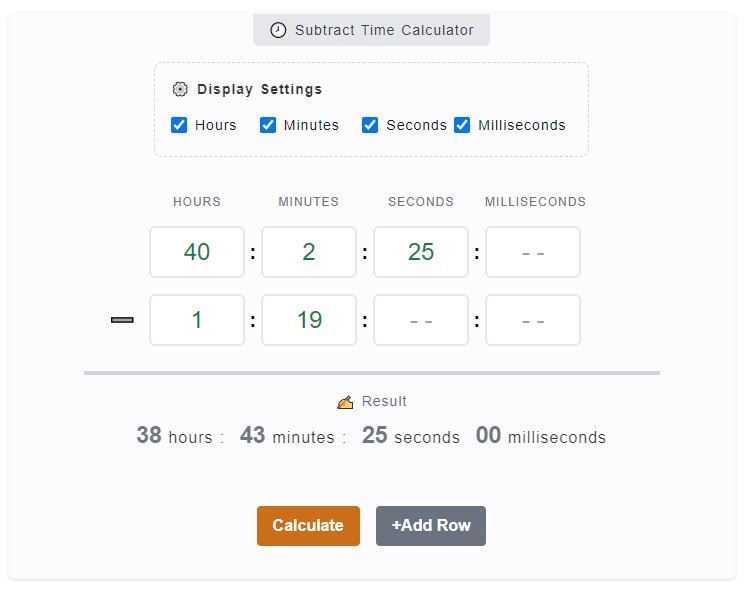# Subtract Time Calculator (with Milliseconds)

The Subtract Time Calculator is a useful tool to obtain the mathematical difference when you subtract a time from another time. So, for example you want to know what is `39 hours and 20 minutes` minus `3 hours and 17 minutes`? You can enter them on the calculator to get the time difference.

🕗 Subtract Time Calculator

### ⚙️ Display Settings

Hours Minutes Seconds MS
: : :
: : :

### ✍ Result

00 hours : 00 minutes :
00 seconds 00 milliseconds

## How to Use the Subtract Time Calculator

The Subtract Time Calculator comes with milliseconds and that useful specially if you are training to a competition and you want to check how fast you have finished an activity than the last one which includes even the milliseconds.

Another situation is you need to work 40 hours a week but you have late deductions like `7 minutes` or `19 minutes`. Since you can have multiple entries on the calculator, you can just quickly enter them up on the calculator to know your total worked hours.1. Step 1

The first thing you might want to do is to check whether you need all hours, minutes, seconds and milliseconds input fields. You can omit the one's you don't need by just unchecking the corresponding checkbox on the `⚙️ Display Settings`.

2. Step 2

The next step is to enter the base time (also called minuend). This is time you want to be subtracted from which is the first row of the time input group. So, for instance, your base or minuend is 56 hours. Just enter `56` on the very first hours input field.

3. Step 3

We already have a minuend or a base time we went to be subtracted from. The next thing to do is to enter the time we want to subtract to or the subtrahend. Following our example above, `56 hours` is our base. If we want to subtract 2 hours, 4 minutes and 28 seconds from that, we can just enter `2` on hours field, `4` on minutes field and `28` on seconds field. Note that these should be entered on the second row of the time group.

4. Step 4

When all the values are entered, you can just click on `Calculate` button to initialize the computation. You will get your result at the bottom of the calculator. Moreover, you can add more subtrahend by just clicking on `+Add Row` button.

## Subtract Time Calculator Inputs and Outputs

The calculator's main goal is simply to give you the mathematical time difference between two (2) time inputs. The calculator looks quiet easy to use but let's make sure that you can use if properly by just mentioning its inputs and outputs.

### Display Settings

The display settings are added on the calculator for you to personalize your inputs. These are in the form of checkboxes, which is checked by default. It includes the `Hours`, `Minutes`, `Seconds` and `Milliseconds`. You can actually uncheck the one's you don't need and it will be hidden on the display.

### Inputs

By default, there are two (2) time input rows on the calculator which includes the hours, minutes, seconds and milliseconds. The first row is where you enter your base time (minuend) while the second row is the time you want to subtract (subtrahend).

### Outputs

The output or the result of the calculation is being displayed right below the input fields which is labeled with `✍ Result`. This shows the mathematical difference of the computation. This calculator includes milliseconds which is really useful for much precise results.

So, what if you have multiple time entries? Either a subtrahend or a minuend, you can enter as much as you want. You can also delete them by clicking on the 🗑️ trash icon. The calculator will do its thing and you'll get a negative time or positive time depending on your inputs.

## How to Subtract Time

The calculator above is an automated approach to quickly getting an answer whenever you want to subtract time. However, you can do it manually by following this guide.

Let's try to subtract `37 hours and 30 minutes` and `3 hours and 12 minutes`.

1.  37 hours `32````0 10``` minutes - 3 hours 1`2` minutes `8` minutes

Let's start doing the first part which is the minutes. Since we cannot subtract 2 from zero, let's borrow 1 from 3, cross that over and make it 2. This is basic math so you're already familiar with this. Now we have 10 - 2 = 8 minutes.

2.  37 hours `32``0 10` minutes - 3 hours `1`2 minutes `1`8 minutes

Moving on to the next digit, since we have borrowed 1 from 3 previously, we now have 2 - 1 = 1 minute. This marks the end of the first part where we are now at 18 minutes difference.

3.  3`7` hours `32``0 10` minutes - `3` hours 12 minutes `5` hours 18 minutes

Let's work now on the hours part. We just simply need to subtract 3 from 7 which will give us the difference of 5. So far we have 5 hours and 18 minutes.

4.  `3`7 hours `32``0 10` minutes - 3 hours 12 minutes `3`5 hours 18 minutes

For the final step since this marks the last digit, there's no subtrahend for 3 so let's just bring that down. Our final answer is `35 hours and 18 minutes`.

## Video Explanation

Here's a video tutorial on how to use the Subract Time Calculator.